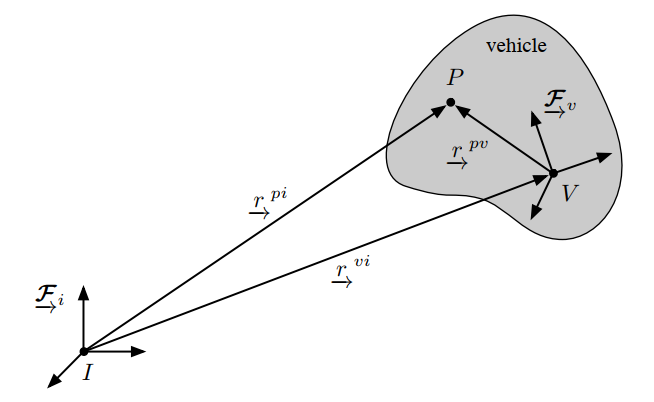https://www.zhe-zhang.com/blog No Title Blog 2020-04-12T06:34:17.246Z https://github.com/jpmonette/feed 一个不想起名的博客 | Too lazy to name it https://www.zhe-zhang.com/blog/images/avatar.png https://www.zhe-zhang.com/blog/favicon.ico All rights reserved 2020, No Title Blog <![CDATA[OpenCV 鱼眼相机模型投影关系]]> https://www.zhe-zhang.com/blog/post/20200409-opencv-fisheye-cam-projection/ 2020-04-09T06:11:02.000Z HighLight:
A detail demo

]]>
HighLight:
A detail demo

## 相机模型

Khmc = np.array([[352.59619801644876, 0.0, 0.0],
[0.0, 352.70276325061578, 0.0],
[654.6810228318458, 400.952228031277, 1.0]]).T
kd = np.array([-0.05631891929412012, -0.0038333424842925286,
-0.00024681888617308917, -0.00012153386798050158])


$\begin{array}{l} a=x / z \text { and } b=y / z \\ r^{2}=a^{2}+b^{2} \\ \theta=\operatorname{atan}(r) \end{array}$

$\theta_{\mathrm{d}}=\theta\left(1+\mathrm{k}_{1} \theta^{2}+\mathrm{k}_{2} \theta^{4}+\mathrm{k}_{3} \theta^{6}+\mathrm{k}_{4} \theta^{8}\right) \\ =\left(\theta+\mathrm{k}_{1} \theta^{3}+\mathrm{k}_{2} \theta^{5}+\mathrm{k}_{3} \theta^{7}+\mathrm{k}_{4} \theta^{9}\right)$

$x' = (\theta_d / r) x \\ y' = (\theta_d / r) y$

]]>

]]>

## A Demo

f=1061
cx=960
cy=540


k = [-0.33, 0.12, -0.022]


$kk = 1 -0.33 r^{2} + 0.12 r^{4} -0.022 r^{6} \tag{1}$

$u = f \cdot (kk \cdot x)+cx \tag{2}$

$u = 1061 \cdot[1 -0.33 x^{2} + 0.12 x^{4} -0.022 x^{6}] \cdot x + 960 \tag{3}$

$u =0$ 时, 3个实根为 $(-1.64, -1.38, 2.23)$;
$u=1920$ 时, 3个实根为 $(-2.23, 1.38, 1.64)$.

## Reference

]]>
<![CDATA[相机参数与基础矩阵笔记]]> https://www.zhe-zhang.com/blog/post/20200323_camera_pose_fundmat/ 2020-03-23T12:59:14.000Z Highligh:

• 详解相机位姿和外参的关系
• 详解基础矩阵(Fundamental Matrix)的计算
]]>
Highligh:

• 详解相机位姿和外参的关系
• 详解基础矩阵(Fundamental Matrix)的计算

## 位姿(Pose)与相机外参$\mathbf{r}_{i}^{p i}=\mathbf{C}_{i v} \mathbf{r}_{v}^{p v}+\mathbf{r}_{i}^{v i} \tag{1}$

$\left\{\mathbf{r}_{i}^{v i}, \mathbf{C}_{i v}\right\}$ 定义为物体$V$在全局坐标系$I$中的位姿.

$x_{C} = R\cdot x_{I} + t \tag{2}$

$\left[\begin{array}{ll} \mathbf{C} & \mathbf{r} \\ \mathbf{0}^{T} & 1 \end{array}\right]^{-1} = \left[\begin{array}{ll} \mathbf{C^{\top}} & \mathbf{-C^{\top}r} \\ \mathbf{0}^{T} & 1 \end{array}\right]$.

(注意: 本文中位姿和外参中旋转和平移分量的位置不同.)

## 基础矩阵(Fundamental Matrix)

(§ 9.2.2 in [2:1])提供了一个示例用于在已知两个相机参数的情况下, 计算其之间基础矩阵.

$\mathrm{F}=\mathrm{K}^{\prime-\top}[\mathbf{t}]_{\times} \mathrm{RK}^{-1} \tag{3}$

$x_{C} = R_{2}R_{1}^{\top}\cdot x_{I^{\prime}} + (t_{2} - R_{2}R_{1}^{\top}t_{1})$

def get_fundmat(cam1, cam2):
"""
Transform cams that cam1's R is identity mat and t is zero vector
$F = \mathrm{K}^{\prime-\top}[\mathrm{t}]_{\times \mathrm{RK}^{-1}}$
1′=Fx
:param cam1:
:param cam2:
:return:
"""
K1 = cam1['K']
K2 = cam2['K']
R1 = cam1['R']
R2 = cam2['R']
t1 = cam1['t']
t2 = cam2['t']
R22 = R2 @ R1.T
t22 = (t2 - R22@t1).reshape(-1)

t_mat = np.zeros((3, 3))  # cross product matrix
t_mat[0, 1] = -t22
t_mat[0, 2] = t22
t_mat[1, 2] = -t22
t_mat = -t_mat.T + t_mat

fundmat = np.linalg.inv(K2).T @ t_mat @ R22 @ np.linalg.inv(K1)
return fundmat


## 参考资料

1. T. D. Barfoot, State Estimation for Robotics. Cambridge: Cambridge University Press, 2017. ↩︎ ↩︎

2. R. Hartley and A. Zisserman, Multiple view geometry in computer vision. 2004. ↩︎ ↩︎

]]>
<![CDATA[Hello Gridea]]> https://www.zhe-zhang.com/blog/post/hello-gridea/ 2018-12-11T16:00:00.000Z 👏 欢迎使用 Gridea
✍️ Gridea 一个静态博客写作客户端。你可以用它来记录你的生活、心情、知识、笔记、创意... ...

]]>
👏 欢迎使用 Gridea
✍️ Gridea 一个静态博客写作客户端。你可以用它来记录你的生活、心情、知识、笔记、创意... ...

## 特性👇

📝 你可以使用最酷的 Markdown 语法，进行快速创作

🌉 你可以给文章配上精美的封面图和在文章任意位置插入图片

🏷️ 你可以对文章进行标签分组

📋 你可以自定义菜单，甚至可以创建外部链接菜单

💻 你可以在 WindowsMacOSLinux 设备上使用此客户端

🌎 你可以使用 𝖦𝗂𝗍𝗁𝗎𝖻 𝖯𝖺𝗀𝖾𝗌Coding Pages 向世界展示，未来将支持更多平台

💬 你可以进行简单的配置，接入 GitalkDisqusJS 评论系统

🇬🇧 你可以使用中文简体英语

🌁 你可以任意使用应用内默认主题或任意第三方主题，强大的主题自定义能力

🖥 你可以自定义源文件夹，利用 OneDrive、百度网盘、iCloud、Dropbox 等进行多设备同步

🌱 当然 Gridea 还很年轻，有很多不足，但请相信，它会不停向前 🏃

😘 Enjoy~

]]>